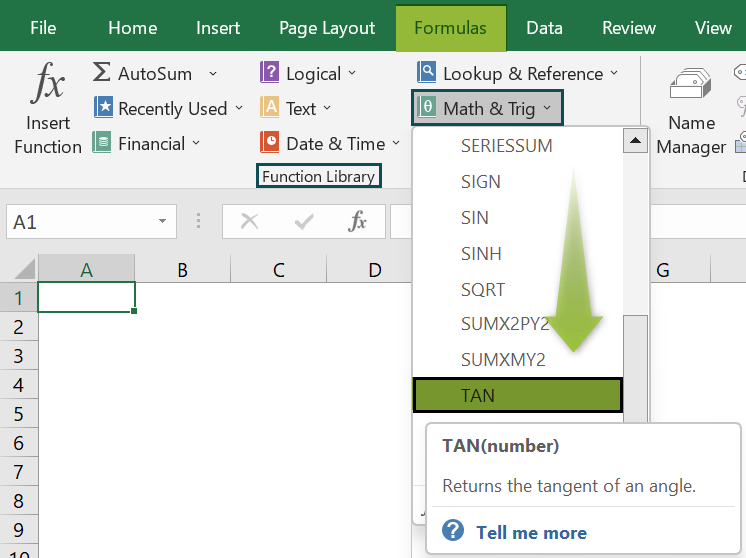# TAN Excel Function [Tangent]Article byExcelMojo Team## What Is TAN Excel Function?

The TANGENT, or TAN Excel function, is a Trigonometric function like COS, SIN, etc. The function helps us to find the tangent, which is based on a right-angle triangle. Firstly, we need to find RADIANS for the given angle, then calculate the TANGENT.

The TAN function in Excel is an inbuilt “Math & Trig” function, so we can insert the formula from the “Function Library” or enter it directly in the worksheet.

For example, the image below depicts the Value in column A. We will apply the TAN formula to calculate the Tangent values as the output.

Select cell B2, enter the formula =TAN(A2 * PI() / 180), and press “Enter”.

The result is ‘1’, as shown above. Column C is for our reference.

###### Key Takeaways
• The TAN Excel function is a trigonometric function that finds the TANGENT of an angle.
• To calculate TAN, we must first calculate the RADIANS using the formula =RADIAN(degree), or we can calculate by multiplying the angle using the expression [PI()/180].
• The TAN function always uses the parameter as the RADIANS function.
• To calculate the TAN value, we use the TAN() function along with other functions such as PI(), RADIANS(), DEGREES(), etc.
• The PI() function helps us derive accurate results while working with π.

### TAN() Excel Formula

The syntax of the TAN() Excel formula is,

The mandatory argument of the TAN() Excel formula is,

• number: It is the angle in radians for which we want the tangent.

### How To Use TAN Excel Function?

We can use the TAN function in 2 ways, namely:

1. Access from the Excel ribbon.
2. Enter in the worksheet manually.

#### Method #1 – Access from the Excel ribbon

Choose an empty cell for the result → select the “Formulas” tab → go to the “Function Library” group → click the “Math & Trig” option drop-down → select the “TAN” function, as shown below.

The “Function Arguments” window appears. Enter the arguments in the “Number” field, and click “OK”, as shown below.

#### Method #2 – Enter in the worksheet manually

1. Select an empty cell for the output.
2. Type =TAN( in the selected cell. [Alternatively, type =T or =TA and double-click the TAN function from the list of suggestions shown by Excel.
3. Enter the argument as cell value or cell reference and close the brackets.
4. Press the “Enter” key.

The succeeding example depicts the values, and we will calculate the Tangent value of the given values using the TAN function combined with the RADIANS function.

In the table, the data is,

• Column A contains the Value.
• Column B contains the Result.

The steps to calculate using the TAN formula are,

1. Select cell B2, and enter the formula =TAN(RADIANS(, i.e., the value of the Radian function.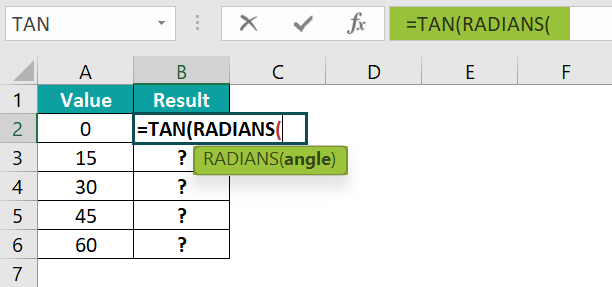2. Enter the value of the ‘angle’ as A2, and close the brackets. The complete formula is =TAN(RADIANS(A2)).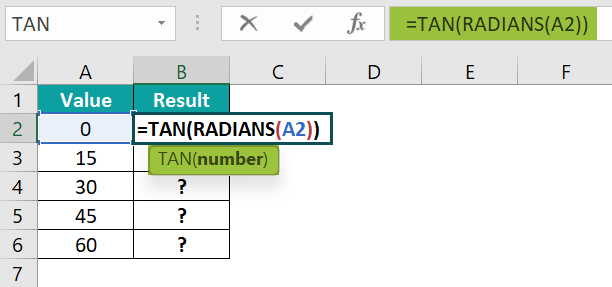3. Press the “Enter” key. The result is “0”, as shown below.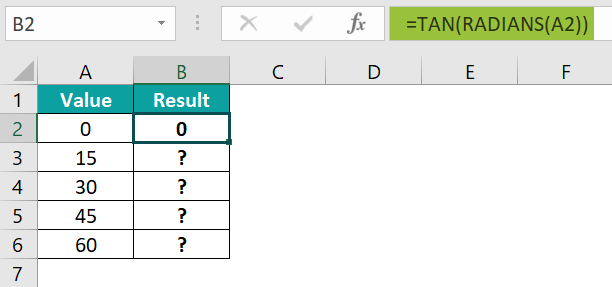4. Drag the formula from cell B2 to B6 using the fill handle. The output is shown below.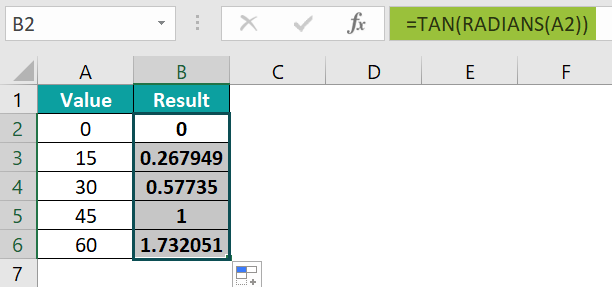### Examples

We will consider some advanced scenarios using the TAN Excel function examples.

#### Example #1

The succeeding example depicts the values, and we will calculate the Tangent value of the given values using the TAN function combined with the RADIANS function.

In the table, the data is,

• Column A contains the Value.
• Column B contains the Result.

The steps to calculate the value using the TAN formula are as follows:

• Step 1: Select cell B2, enter the formula =TAN(RADIANS(A2)), and press the “Enter” key. The result is “1.63246E+16”, as shown below.
• Step 2: Drag the formula from cell B2 to B5 using the fill handle. The output is shown below.

#### Example #2

The succeeding example depicts the values, and we will calculate the Tangent value of the given values using the TAN function combined with the DEGREES function.

In the table, the data is,

• Column A contains the Value.
• Column B contains the Result.

The steps to calculate the value using the TAN formula are as follows:

• Step 1: Select cell B2, enter the formula =TAN(DEGREES(A2)), and press the “Enter” key. The result is “0”, as shown below.
• Step 2: Drag the formula from cell B2 to B6 using the fill handle. The output is shown below.

#### Example #3

We will consider the same data from Example 1, which depicts the values, and we will calculate the Tangent value of the given values using the TAN function combined with the DEGREES function.

In the table, the data is,

• Column A contains the Value.
• Column B contains the Result.

The steps to calculate the value using the TAN formula are as follows:

• Step 1: Select cell B2, enter the formula =TAN(DEGREES(A2)), and press the “Enter” key. The result is “3.185450512”, as shown below.
• Step 2: Drag the formula from cell B2 to B5 using the fill handle. The output is shown below.

#### Example #4

The succeeding example depicts the values, and we will calculate the Tangent value of the given values using the TAN Excel function combined with the PI in excel and RADIANS functions.

In the table, the data is,

• Column A contains the Value.
• Column B contains the Result.
• Column C contains the Formula.

The procedure to calculate the value using the TAN formula are as follows:

Enter the formulas as shown below,

• =TAN(PI()) in cell B2,
• =TAN(PI() /2) in cell B3,
• =TAN(A2 * PI() / 180) in cell B4,
• =TAN(RADIANS(A2)) in cell B5 and
• Press the “Enter” key after entering each formula.

The results are shown in the image below from cell B2 to B5. Column C is for our reference.

### Important Things To Note

• We get the “#VALUE!” error when the selected cell value or cell reference is non-numeric.
• If the cell chooses a blank cell in the place of a number, the result would be one because the tangent is 1 for the radiant 0.
• We get the “#NAME?” error when the function name is incorrect.

1. What does the TAN function do in Excel?

The TAN function calculates the tangent of a given angle.
The syntax of the TAN function is = TAN(number)

2. How does the TAN function work?

The Excel TAN Function works in Excel as follows:
1) Select an empty cell for the output.
2) Type =TAN( in the selected cell. [Alternatively, type =T or =TA and double-click the TAN function from the list of suggestions shown by Excel.
3) Enter the argument as cell value or cell reference and close the brackets.
4) Press the “Enter” key.

For example, the image depicts the values, and we will calculate the Tangent value of the given values using the TAN Excel function.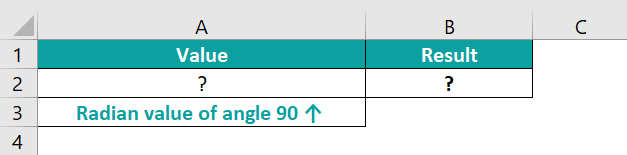The procedure to calculate the value using the TAN formula is,

First, select cell A2, enter the formula =RADIANS(90), and press “Enter”. The result is “1.570796327”, as shown below.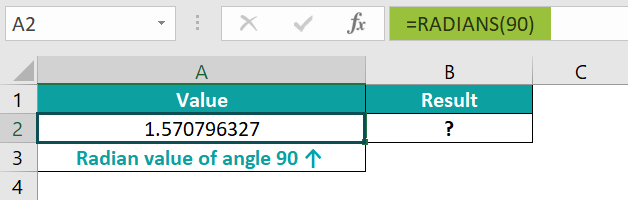Next, select cell B2, enter the formula =TAN(A2), and press “Enter”. The result is “1.63246E+16”, as shown below.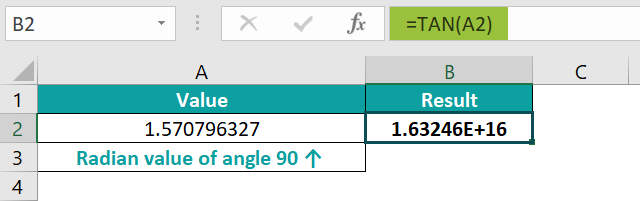3. Where is the TAN function in Excel?

The TAN function in Excel is in the “Formulas” tab, as shown below.

Choose an empty cell for the result → select the “Formulas” tab → go to the “Function Library” group → click the “Math & Trig” option drop-down → select the “TAN” function, as shown below.